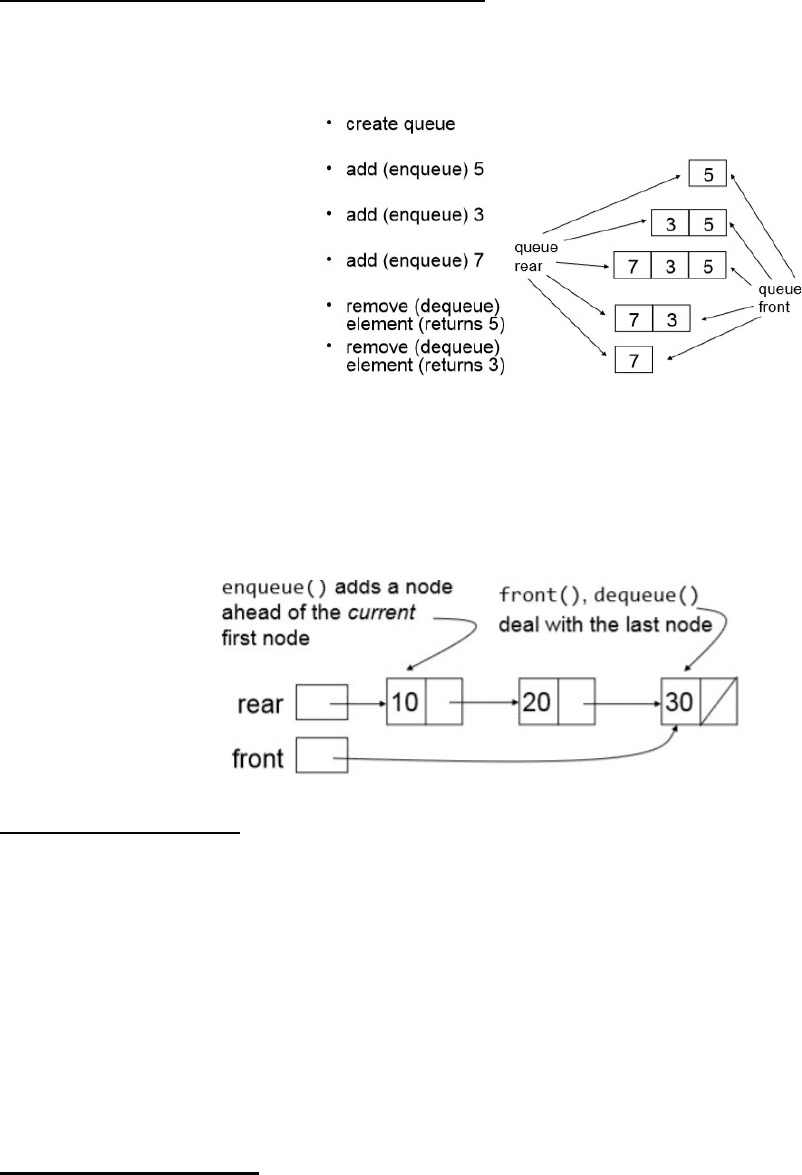# SYSC 2006 Lecture Notes - Lecture 4: C Dynamic Memory Allocation, Linked List

113 views6 pagesImplementing a Queue with a Linked List:
A queue is a collection in which the elements are maintained in the order in which they were added
A queue is a first
-
in, first out (FIFO) collection
-
the first element is the first one retrieved or removed
Try removing/replacing a node from middle of queue or from rear of queue
Inserting a node anywhere (rear allowed only)
Since they are last in, first out queues do not:
Dequeue means to remove the node at the front of the list
Structure Definition:
typedef struct {
intnode_t *front;
intnode_t *rear;
int size;
} intqueue_t;
Front points to the node at the head of the queue's linked list.
Rear points to the node at the tail of the queue's linked list.
Size contains the # of nodes in the queue's linked list.
Constructing a Queue:
intqueue_t *intqueue_construct(void)
{
intqueue_t *queue = malloc(sizeof(intqueue_t));
// assert(queue != NULL);
Queues
May 4, 2018
4:46 PM
New Section 1 Page 1
Unlock document

This preview shows pages 1-2 of the document.
Unlock all 6 pages and 3 million more documents.// assert(queue != NULL);
queue
-
>front = NULL;
queue
-
>rear = NULL;
queue
-
>size = 0;
return queue;
}
This returns a pointer to a new, empty queuewhich is created in malloc and has the structure of a
Creating a Node in a Queue (inserting at the rear):
void intqueue_enqueue(intqueue_t *queue, int value)
{
//assert(queue != NULL);
intnode_t *p = intnode_construct(value, NULL);
if (queue
-
>front == NULL) {
queue
-
>front = p;
} else {
queue
-
>rear
-
>next = p;
}
queue
-
>rear = p;
queue
-
>size += 1;
}
See Previous notes for intnode construct function and it's structure definition
P points to a new node containing the value to be added and NULL (created in Heap)
If the queue is empty, the front points to p otherwise the next member of the last node points to p
Then the rear member is pointed to p since it always points to the newest node added
Size is increased
_Bool intqueue_dequeue(intqueue_t *queue, int *element)
{
// assert(queue != NULL);
if (queue
-
>size == 0) {
return false;
}
*element = queue
-
>front
-
>value;
intnode_t *node_to_delete = queue
-
>front;
queue
-
>front = queue
-
>front
-
>next;
free(node_to_delete);
Deleting the node at the front and returning that node's value :
New Section 1 Page 2
Unlock document

This preview shows pages 1-2 of the document.
Unlock all 6 pages and 3 million more documents.

## Document Summary

A queue is a collection in which the elements are maintained in the order in which they were added. A queue is a first-in, first out (fifo) collection - the first element is the first one retrieved or removed. Since they are last in, first out queues do not: Try removing/replacing a node from middle of queue or from rear of queue. Enqueue means to insert a node at the rear. Dequeue means to remove the node at the front of the list. Structure definition: typedef struct { intnode_t *front; intnode_t *rear; int size; Front points to the node at the head of the queue"s linked list. Rear points to the node at the tail of the queue"s linked list: size contains the # of nodes in the queue"s linked list. Constructing a queue: intqueue_t *intqueue_construct(void) intqueue_t *queue = malloc(sizeof(intqueue_t)); // assert(queue != null); queue->front = null; queue->rear = null; queue->size = 0; return queue;

## Get access

\$8 USD/m\$10 USD/m
Billed \$96 USD annuallyHomework Help
Study Guides
Textbook Solutions
Class Notes
Textbook Notes
Booster Class